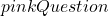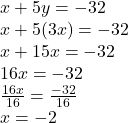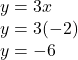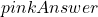solve this system using substitution x+5y=-32 y=3x

Question

solve this system using substitution x+5y=-32 y=3x

in progress 0
6 months 2021-07-16T11:54:39+00:00 1 Answers 2 views 0

1.solve this system using substitution x+5y=-32 y=3x

Substitute 3x for y in x + 5y = – 32Substitute – 2 for for x in y = 3xy = – 6

Hope it helps ^_^